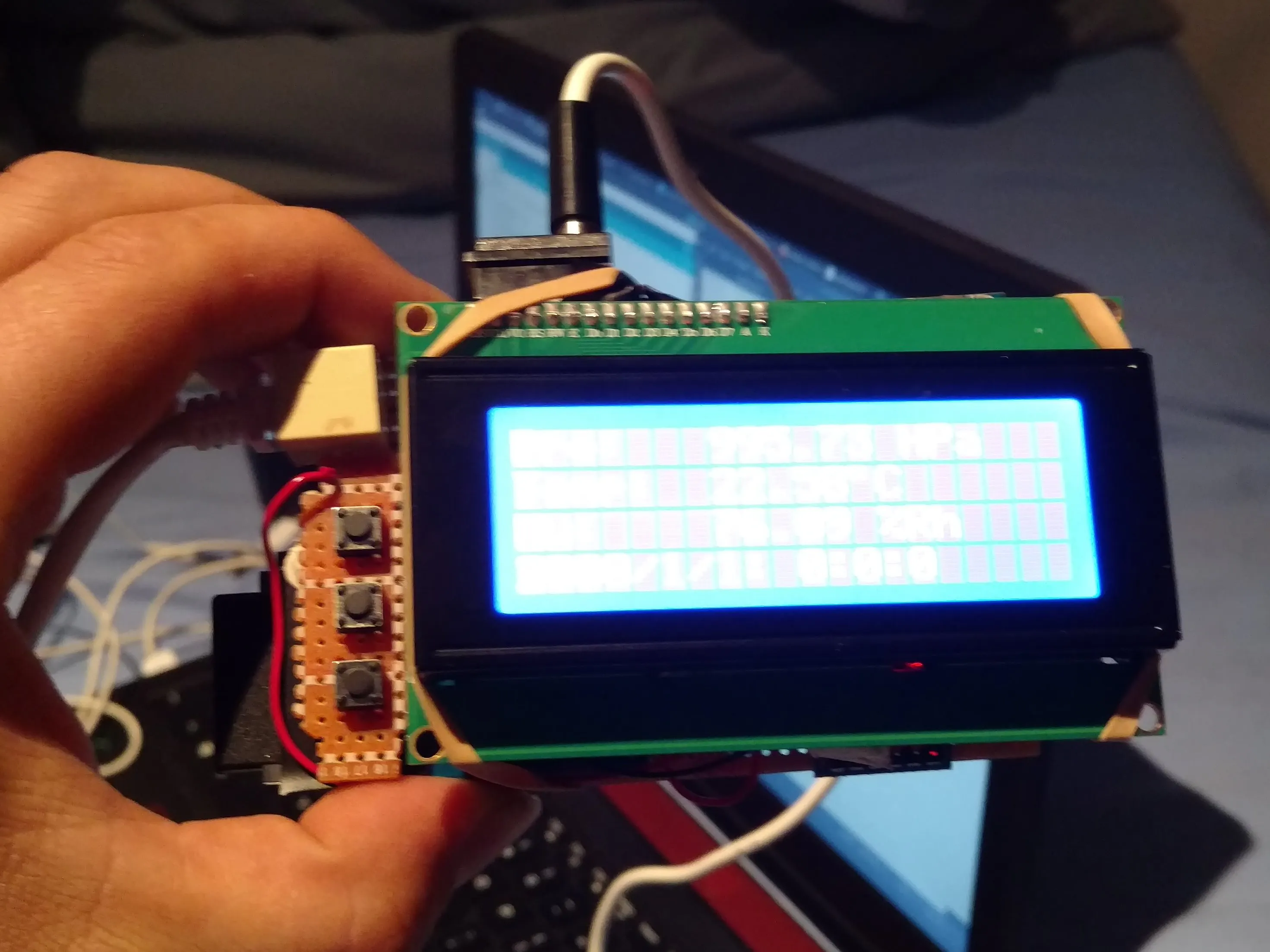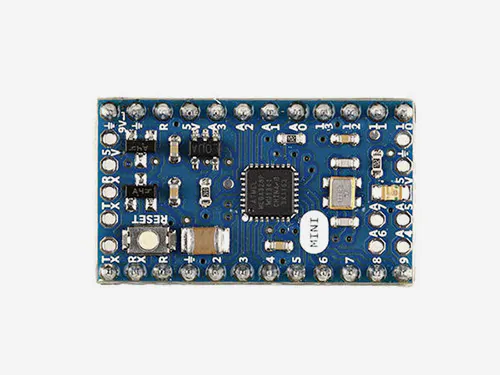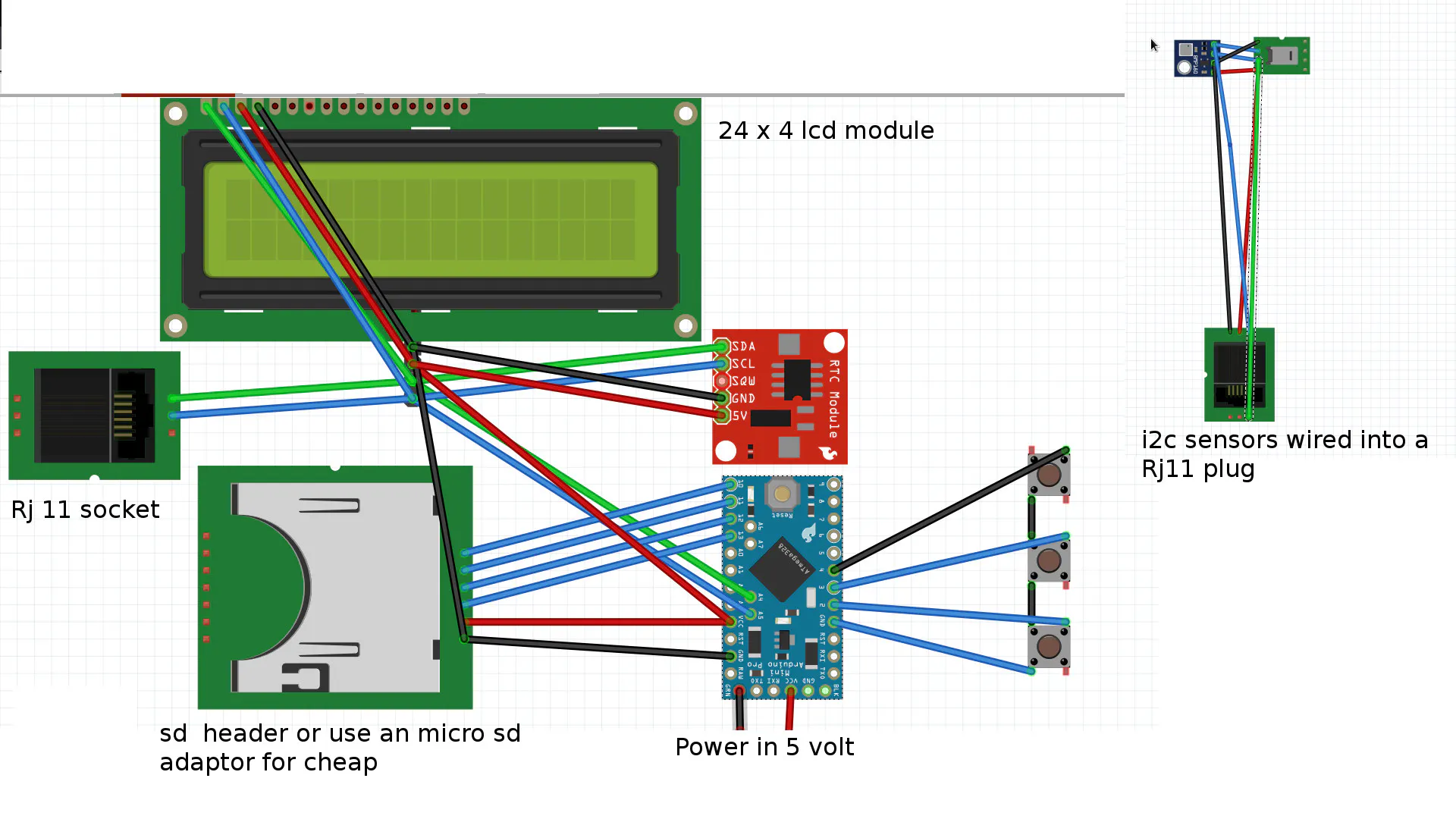# Simple Mini Logger

A simple humidity, temperature, and pressure data logger.

IntermediateShowcase (no instructions)5 hours2,876## Things used in this project

### Hardware componentsArduino Mini 05
×1
 sht31-d
×1
 bmp180
×1
 rtc module
×1
 sd card module
×1
 rj 11 socket
×1
×1
 50k potentiometer
×1

## Schematics

### Micro-logger

Its a small simple humidity temperature pressure logger with time## Code

### Micro_logger

Arduino
```#include <Wire.h>
#include <LiquidCrystal_I2C.h>
#include <WEMOS_SHT3X.h>
#include "RTClib.h"
#include "DS1307.h"
#include <SPI.h>
#include <SD.h>
#define TEMPERATURE_PRECISION 14

const int chipSelect = 10;

LiquidCrystal_I2C lcd(0x27, 2, 1, 0, 4, 5, 6, 7, 3, POSITIVE);  // Set the LCD I2C address
SHT3X sht30(0x45);        // SHT30 object to enable readings
RTC_DS1307 rtc;
DS1307 clock;//define a object of DS1307 class

char ascii=0x00+223;
float TE = 0.1;
float HU = 0.1;
const int buttonPin = 2;
int buttonState = HIGH;         // variable for reading the pushbutton status

void setup() {

const int buttonPin = 2;
const int buttonPin1 = 4;
const int buttonPin2 = 3;
int p = 2018;
int l = 0;
int j = 0;
int h = 0;
int m = 0;
int buttonState = HIGH;
int buttonState1 = HIGH;
int buttonState2 = HIGH;

lcd.begin(20,4);   // initialize the lcd for 16 chars 2 lines, turn on backlight
lcd.noCursor();
pinMode(buttonPin, INPUT);
digitalWrite(buttonPin, HIGH);

if (buttonState == 0) { // if the button is presed while turning on device it enters  the set time menu
lcd.print("SET");
delay(1000);
clock.begin();
DateTime now = rtc.now();

pinMode(buttonPin2, INPUT);
digitalWrite(buttonPin2, HIGH);

pinMode(buttonPin1, INPUT);
digitalWrite(buttonPin1, HIGH);
lcd.clear();
while (buttonState == 1){
lcd.print("Year: ");
lcd.print(p);
lcd.setCursor(0,3);
lcd.print("Next");
delay(100);
lcd.setCursor(0,0);

if (buttonState1 == 0){
p = p + 1;
delay(100);}

if (buttonState2 == 0){
p = p - 1;
delay(100);}
}

lcd.clear();
delay(500);

while (buttonState == 1){
lcd.print("Month: ");
lcd.print(l);
lcd.setCursor(0,3);
lcd.print("Next");
delay(100);
lcd.setCursor(8,0);
lcd.print("  ");
lcd.setCursor(0,0);

if (buttonState1 == 0){
l = l + 1;
delay(100);}

if (buttonState2 == 0){
l = l - 1;
delay(100);}
}
lcd.clear();
delay(500);

while (buttonState == 1){
lcd.print("Day: ");
lcd.print(j);
lcd.setCursor(0,3);
lcd.print("Next");
delay(100);
lcd.setCursor(6,0);
lcd.print("  ");
lcd.setCursor(0,0);

if (buttonState1 == 0){
j = j + 1;
delay(100);}

if (buttonState2 == 0){
j = j - 1;
delay(100);}
}
lcd.clear();
delay(500);

while (buttonState == 1){
lcd.print("Hour:  ");
lcd.print(h);
lcd.setCursor(0,3);
lcd.print("Next");
delay(100);
lcd.setCursor(8,0);
lcd.print("  ");
lcd.setCursor(0,0);

if (buttonState1 == 0){
h = h + 1;
delay(100);}

if (buttonState2 == 0){
h = h - 1;
delay(100);}
}
lcd.clear();
delay(500);

while (buttonState == 1){
lcd.print("Minute: ");
lcd.print(m);
lcd.setCursor(0,3);
lcd.print("Next");
delay(100);
lcd.setCursor(9,0);
lcd.print("  ");
lcd.setCursor(0,0);

if (buttonState1 == 0){
m = m + 1;
delay(100);}

if (buttonState2 == 0){
m = m - 1;
delay(100);}
}

clock.fillByYMD(p,l,j);
clock.fillByHMS(h,m,0);
clock.setTime();//write time to the RTC chip
}                                              /// end of set time menu
clock.begin();
DateTime now = rtc.now();

Wire.begin();
rtc.begin();
lcd.begin(20,4);   // initialize the lcd for 16 chars 2 lines, turn on backlight
lcd.noCursor();
lcd.print(buttonState);
delay(1000);

bmp.begin();

}

void loop()
{

int n = 0;

n = n + 1;
DateTime now = rtc.now();
sht30.get();
float dew_point = 243.04*(log(sht30.humidity/100)+((17.625*sht30.fTemp)/(243.04+sht30.fTemp)))/(17.625-log(sht30.humidity/100)-((17.625*sht30.fTemp)/(243.04+sht30.fTemp)));
float RHx       = sht30.humidity;
float T         = sht30.fTemp;
float heat_index;
heat_index =(-42.379+(2.04901523*T)+(10.14333127*RHx)-(0.22475541*T*RHx)-(0.00683783*T*T)-(0.05481717*RHx*RHx)+(0.00122874*T*T*RHx)+(0.00085282*T*RHx*RHx)-(0.00000199*T*T*RHx*RHx)-32)*5/9;
if ((sht30.cTemp <= 26.66) || (sht30.humidity <= 40)) heat_index = sht30.cTemp;
float PC = P /100;
TE = sht30.cTemp;
HU = sht30.humidity;
lcd.setCursor(0,0);
lcd.print("Pre:  "); lcd.print("      "); lcd.setCursor(7,0); lcd.print(PC); lcd.print(" HPa");

lcd.setCursor(0,1);
lcd.print("Temp: "); lcd.print("      "); lcd.setCursor(7,1); lcd.print(TE); lcd.print(ascii);lcd.print("C");
lcd.setCursor(0,2);
lcd.print("Hu:   "); lcd.print("      "); lcd.setCursor(7,2); lcd.print(HU);lcd.print(" %Rh");
lcd.setCursor(17,3);
lcd.print("   ");
lcd.setCursor(0,3);
lcd.setCursor(0,3);
lcd.print(now.year(), DEC);
lcd.print('/');
lcd.print(now.month(), DEC);
lcd.print('/');
lcd.print(now.day(), DEC);
lcd.print(": ");
lcd.print(now.hour(), DEC);
lcd.print(':');
lcd.print(now.minute(), DEC);
lcd.print(':');
lcd.print(now.second(), DEC);

int Ti = now.second();

xif (Ti < 1){
DateTime now = rtc.now();

float dew_point = 243.04*(log(sht30.humidity/100)+((17.625*sht30.fTemp)/(243.04+sht30.fTemp)))/(17.625-log(sht30.humidity/100)-((17.625*sht30.fTemp)/(243.04+sht30.fTemp)));
float RHx       = sht30.humidity;
float T         = sht30.fTemp;
float heat_index;
heat_index =(-42.379+(2.04901523*T)+(10.14333127*RHx)-(0.22475541*T*RHx)-(0.00683783*T*T)-(0.05481717*RHx*RHx)+(0.00122874*T*T*RHx)+(0.00085282*T*RHx*RHx)-(0.00000199*T*T*RHx*RHx)-32)*5/9;
if ((sht30.cTemp <= 26.66) || (sht30.humidity <= 40)) heat_index = sht30.cTemp;
float PC = P /100;

String dataString = "";
float b = (sht30.cTemp,2);
float c = (sht30.humidity,2);
dataString += String(now.year(), DEC);
dataString += String('/');
dataString += String(now.month(), DEC);
dataString += String('/');
dataString += String(now.day(), DEC);
dataString += String(": ");
dataString += String(now.hour(), DEC);
dataString += String(':');
dataString += String(now.minute(), DEC);
dataString += String(':');
dataString += String(now.second(), DEC);
dataString += ",";

dataString += String(PC);
dataString += ",";
dataString += TE;
dataString += ",";
dataString += HU;

SD.begin(10);
// open the file. note that only one file can be open at a time,
// so you have to close this one before opening another.
File dataFile = SD.open("datalog.txt", FILE_WRITE);

// if the file is available, write to it:
if (dataFile) {
dataFile.println(dataString);
dataFile.close();
// print to the serial port too:

}
// if the file isn't open, pop up an error:
else {
lcd.println("error opening datalog.txt");
delay(500);
lcd.clear();
}
}
}
```

## Credits

### matthew Champion

4 projects • 4 followers
Llandovery, Wales